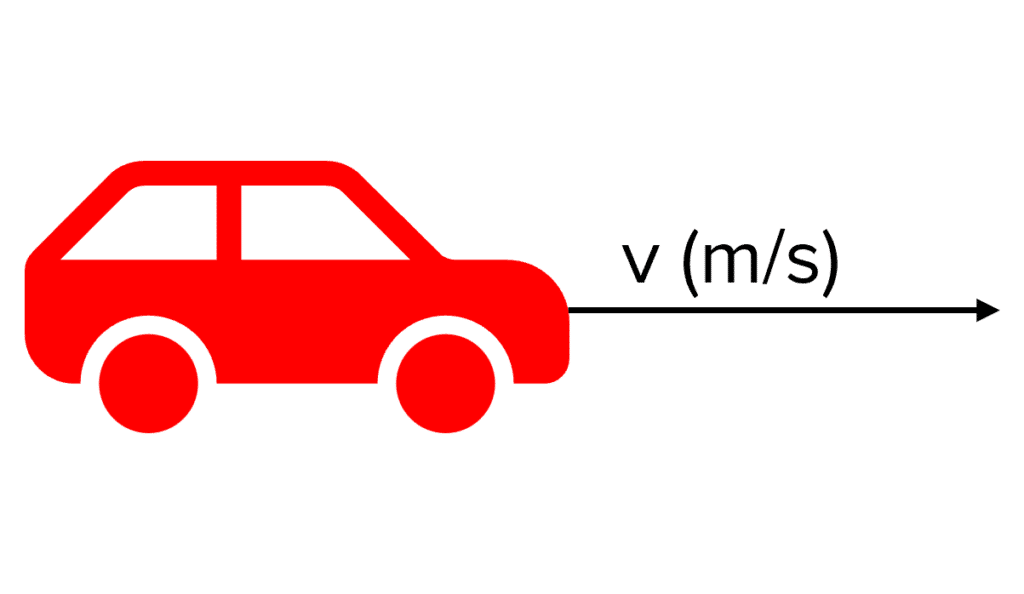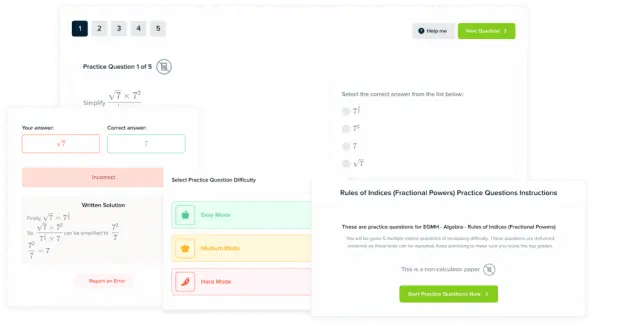# Power

GCSEAQACombined Science FoundationCombined Science HigherPhysics FoundationPhysics Higher

## Power

Power is a way to describe how quickly energy is transferred from one store to another or one object to another. For example, the power of your kettle tells you how quickly electrical energy is converted to thermal energy to heat up the water.

## Power Definitions and Equations

The definition of power is: the rate at which energy is transferred.

This is the same as saying: the rate at which work is done.

Power is measured in Watts $\text{(W)}$. One Watt is equal to $1$ joule $\text{(J)}$ of energy transfer (or work done) per second $\text{s}$

Power can be calculated using either of the following equations:

$P=\dfrac{E}{t} P=\dfrac{W}{t}$

• $P =$ Power $\text{(W)}$
• $E =$ Energy transferred $\text{(J)}$
• $W=$ Work done $\text{(J)}$
• $t =$ time $\text{(s)}$
GCSECombined Science FoundationCombined Science HigherPhysics FoundationPhysics HigherAQA

## Comparing Power

If you know the power of two otherwise identical machines, you can deduce how quickly they use energy and therefore compare their performance.

For example, microwave oven A has two times the power of microwave oven B. This means that microwave oven A transfers energy into food two times faster than microwave oven B. So, you only have to cook the food for half the time

Another example is two cyclists. One cyclist is three times more powerful than the other. The more powerful cyclist does work on the bicycle three times faster and therefore travels at three times the speed of the other cyclist.

GCSECombined Science FoundationCombined Science HigherPhysics FoundationPhysics HigherAQA

## Example 1: Calculating PowerA car is driving at a constant velocity along a flat road in a $100\text{ m}$ race.

a) The car uses $50 000\text{ J}$ whilst driving forwards for $10\text{ s}$. Calculate the power of the car’s engine

[2 marks]

$P=\dfrac{E}{t}=\dfrac{\textcolor{123196}{50 000}}{\textcolor{00bfa8}{10}}=\bold{5 000\text{ W}}$

b) If a different car with a higher power engine uses the same amount of energy, which care will finish the race first? Assume that both cars are identical except for the engine.

[1 mark]

The higher power car will finish the race first. The engine transfers more energy to kinetic energy in the same time and so the car travels faster.

GCSECombined Science FoundationCombined Science HigherPhysics FoundationPhysics HigherAQA

## Example 2: Calculating Power Using Work Done

It takes $50 \text{ kJ}$ of work for a crane to lift a storage container and place it on top of another. The storage container is in the air for $50 \text{ s}$, calculate the power of the crane. State the units of your answer.

[3 marks]

\begin{aligned}\bold{P} &=\bold{\dfrac{W}{t}} \\ &= \dfrac{\textcolor{f21cc2}{50 000 \text{ J}}}{\textcolor{aa57ff}{50 \text{ s}}}\\ &= \bold{1000} \textbf{ W} \end{aligned}

GCSECombined Science FoundationCombined Science HigherPhysics FoundationPhysics HigherAQA

## Power Example Questions

$P=\bold{\dfrac{E}{t}} = \dfrac{150}{30} = \bold{5 \text{ W}}$Gold Standard Education

$P=\bold{\dfrac{W}{t}} = \dfrac{10}{0.5} = \bold{20\text{ W}}$Gold Standard Education

Bulb B is brighter than bulb A.

Because bulb B has a higher power, energy is transferred into light faster in bulb B and so it is brighter.Gold Standard EducationProduct